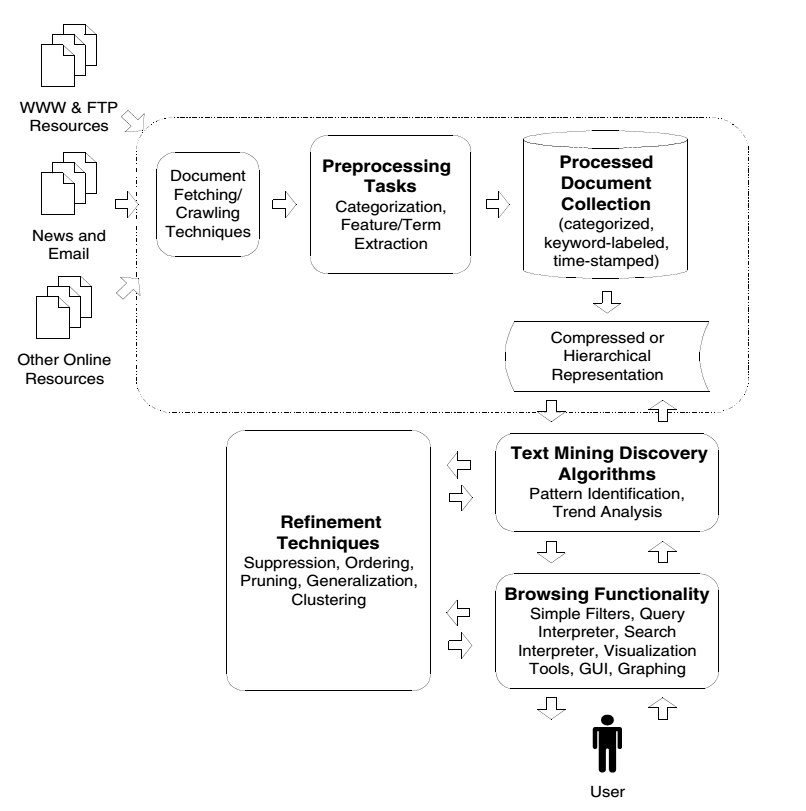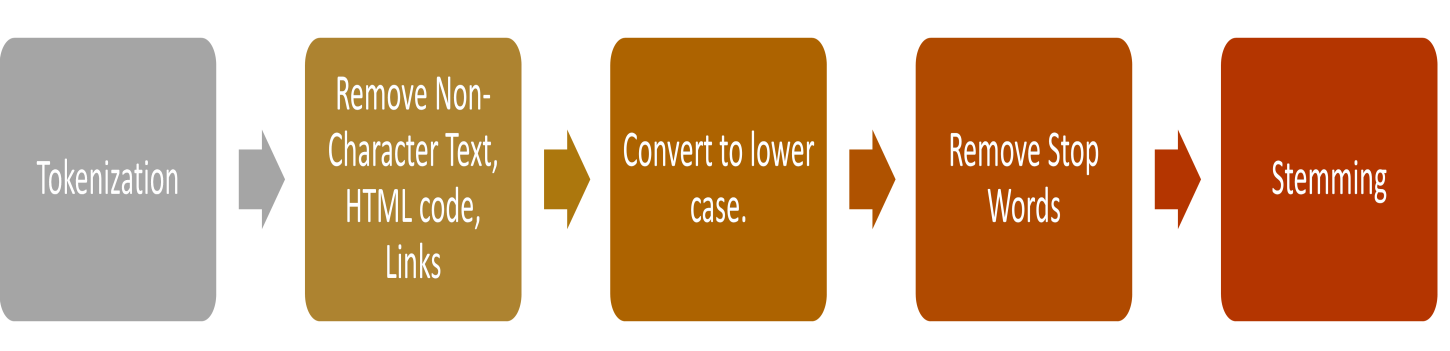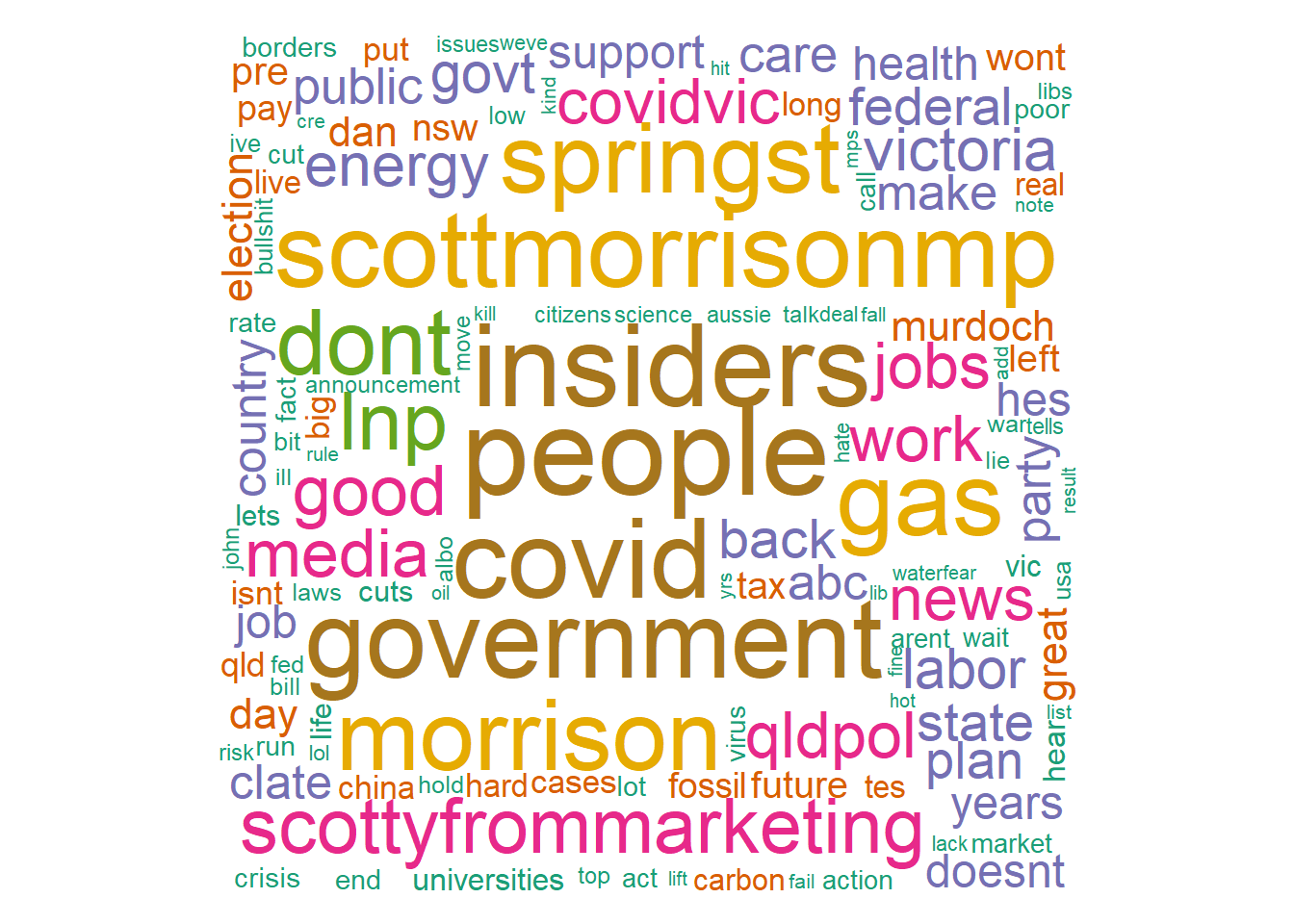# Topic 17 Text Mining using R“We Facebook users have been building a treasure lode of big data that government and corporate researchers have been mining to predict and influence what we buy and for whom we vote. We have been handing over to them vast quantities of information about ourselves and our friends, loved ones and acquaintances”-Douglas Rushkoff

## 17.1 Introduction to Text Mining

Text mining, also referred to as text data mining, roughly equivalent to text analytics, refers to the process of deriving high-quality information from text. High-quality information is typically derived through the devising of patterns and trends through means such as statistical pattern learning.

• Welbers et al. (2017) provides a mild introduction to Text Analytics using R

• Text mining has gained momentum and is used in analytics worldwide

• Sentiment Analysis

• Predicting Stock Market and other Financial Applications

• Customer influence

• News Analytics

• Social Network Analysis

• Customer Service and Help Desk

### 17.1.1 Text Data

• Text data is ubiquitous in social media analytics.

• Traditional media, social media, survey data, and numerous other sources.

• Massive quantity of text in the modern information age.

• The mounting availability of and interest in text data has been the development of a variety of statistical approaches for analysing this data.

### 17.1.2 Generic Text Mining System

• Following figure demonstrates a general text mining system (Source: Feldman & Sanger (2007))
knitr::include_graphics("fig-2.png")Figure 17.1: Generic Text Mining System

### 17.1.3 Data pre-processing in Text Mining

• Following figure summarises main steps in a typical data pre-processing stage of text mining
knitr::include_graphics("fig-3.png")Figure 17.2: Typical Text Pre-procesing

## 17.2 Mining Twitter Text Data using R

Twitter is one of the most popular social media platform for information sharing. Some examples from rforresearch tweets rforresearch?)

• Twitter provides an API access for their data feed.

• User is required to create an app and obtain access codes for the API.

• See here https://cran.r-project.org/web/packages/rtweet/vignettes/auth.html for a detailed introduction on obtaining API credentials.

• Data access is limited in various ways (days, size of data etc.).

• See here https://developer.twitter.com/en/docs.html for full documentation.

• R provides packages like twitteR and rtweet provide programming interface using R to access the data.

• Twitter doesnt allow data sharing but the API setup is straight forward. Twitter does allow sharing of the tweet IDs (available in the data folder)

• We will download tweets with ‘#auspol’; a popular hashtag used in Australia to talk about current affairs and socio-political issues
• After setting up the API
• Create the token
• search_tweets can be used without creating the token, it will create a token using the default app for your Twitter account
library(rtweet)

token <- create_token(app = "your_app_name", consumer_key = "your_consumer_key",
consumer_secret = "your_consumer_secret", access_token = "your_access_token",
access_secret = "your_access_secret")
• Use the search_tweets function to download the data, convert to data frame and save
rt = search_tweets(q = "#auspol", n = 15000, type = "recent", include_rts = FALSE,
since = "2020-09-14")
rt2 = as.data.frame(rt)
saveRDS(rt2, file = "tweets_auspol.rds")

## 17.4 Data Pre-processing

• We will conduct data pre-processing in this stage

• Some steps (depend on the types of problems analysed)

• Create a corpus
• Change encoding
• Convert to lower case
• Remove hashtags
• Remove URLs
• Remove @ mentions
• Remove punctuations
• Remove stop words
• Stemming can also be conducting (avoided in this example)
library(tm)
# encoding
rt$text <- sapply(rt$text, function(row) iconv(row, "latin1", "ASCII", sub = ""))

# build a corpus, and specify the source to be character vectors

myCorpus = Corpus(VectorSource(rt$text)) # convert to lower case myCorpus = tm_map(myCorpus, content_transformer(tolower)) # remove punctuation myCorpus <- tm_map(myCorpus, removePunctuation) # remove numbers myCorpus <- tm_map(myCorpus, removeNumbers) # remove URLs removeURL = function(x) gsub("http[^[:space:]]*", "", x) removeURLs = function(x) gsub("https[^[:space:]]*", "", x) # remove hashtags removehash = function(x) gsub("#\\S+", "", x) # remove @ removeats <- function(x) gsub("@\\w+", "", x) #only removes the '@' # remove numbers and punctuations removeNumPunct = function(x) gsub("[^[:alpha:][:space:]]*", "", x) # leading and trailing white spaces wspace1 = function(x) gsub("^[[:space:]]*", "", x) ## Remove leading whitespaces wspace2 = function(x) gsub("[[:space:]]*$", "", x)  ## Remove trailing whitespaces
wspace3 = function(x) gsub(" +", " ", x)  ## Remove extra whitespaces

removeIms <- function(x) gsub("im", "", x)

myCorpus = tm_map(myCorpus, content_transformer(removeURL))  #url
myCorpus = tm_map(myCorpus, content_transformer(removeURLs))  #url

myCorpus <- tm_map(myCorpus, content_transformer(removehash))  #hashtag
myCorpus <- tm_map(myCorpus, content_transformer(removeats))  #mentions

myCorpus = tm_map(myCorpus, content_transformer(removeNumPunct))  #number and punctuation (just in case some are left over)

myCorpus = tm_map(myCorpus, content_transformer(removeIms))  #Ims

myCorpus = tm_map(myCorpus, content_transformer(wspace1))
myCorpus = tm_map(myCorpus, content_transformer(wspace2))
myCorpus = tm_map(myCorpus, content_transformer(wspace3))  #other white spaces

# remove extra whitespace
myCorpus = tm_map(myCorpus, stripWhitespace)

# remove extra stopwords
myStopwords = c(stopwords("English"), stopwords("SMART"), "rt", "ht", "via", "amp",
"the", "australia", "australians", "australian", "auspol")

myCorpus = tm_map(myCorpus, removeWords, myStopwords)

# generally a good idea to save the processed corpus now
save(myCorpus, file = "auspol_sep.RData")

data_tw2 = data.frame(text = get("content", myCorpus), row.names = NULL)
data_tw2 = cbind(data_tw2, ID = rt$status_id) data_tw2 = cbind(Date = as.Date(rt$created_at), data_tw2)
# look at the data frame, still some white spaces left so let's get rid of them
data_tw2$text = gsub("\r?\n|\r", "", data_tw2$text)
data_tw2$text = gsub(" +", " ", data_tw2$text)
# save data
saveRDS(data_tw2, file = "processed_data.rds")

## 17.5 Some Visualisation

• Bar Chart of top words
library(tm)
# Build TDM
tdm = TermDocumentMatrix(myCorpus, control = list(wordLengths = c(3, Inf)))
m = as.matrix(tdm)
word.freq = sort(rowSums(m), decreasing = T)
# plot term freq
term.freq1 = rowSums(as.matrix(tdm))
term.freq = subset(term.freq1, term.freq1 >= 50)
df = data.frame(term = names(term.freq), freq = term.freq)
df = transform(df, term = reorder(term, freq))
library(ggplot2)
library(ggthemes)

m2 = ggplot(head(df, n = 20), aes(x = reorder(term, -freq), y = freq)) + geom_bar(stat = "identity",
aes(fill = term)) + theme(legend.position = "none") + ggtitle("Top 20 words in tweets #auspol \n (14 Sep to 20 Sep 2020)") +
theme(axis.text = element_text(size = 12, angle = 90, face = "bold"), axis.title.x = element_blank(),
title = element_text(size = 15))
m2 = m2 + xlab("Words") + ylab("Frequency") + theme_wsj() + theme(legend.position = "none",
text = element_text(face = "bold", size = 10))
m2Figure 17.3: Top 20 Words

• Word Cloud 1
library(wordcloud)
library(RColorBrewer)
pal = brewer.pal(7, "Dark2")
wordcloud(words = names(word.freq), freq = word.freq, min.freq = 5, max.words = 1000,
random.order = F, colors = pal)Figure 17.4: Wordcloud-1

• Word Cloud 2
library(wordcloud2)
# some data re-arrangement
term.freq2 = data.frame(word = names(term.freq1), freq = term.freq1)
tw2 = data_sent$text • Conduct Sentiment Analysis and Visualise mySentiment = get_nrc_sentiment(tw2) #this can take some time tweets_sentiment = cbind(data_sent, mySentiment) # save the results saveRDS(tweets_sentiment, file = "tw_sentiment.rds") • Plot sentimentTotals = data.frame(colSums(tweets_sentiment[, c(4:13)])) names(sentimentTotals) = "count" sentimentTotals = cbind(sentiment = rownames(sentimentTotals), sentimentTotals) rownames(sentimentTotals) = NULL # plot ggplot(data = sentimentTotals, aes(x = sentiment, y = count)) + geom_bar(aes(fill = sentiment), stat = "identity") + theme(legend.position = "none") + xlab("Sentiment") + ylab("Total Count") + ggtitle("Sentiment Score for Sample Tweets") + theme_minimal() + theme(axis.text = element_text(size = 15, face = "bold"))Figure 17.7: Emotions/Sentiment Scores ## 17.7 Topic Modelling This section will use bi-term topic modelling method to demonstrate topic modelling exercise. Biterm Topic Modelling (BTM) (Yan, Guo, Lan, & Cheng (2013)) is useful for short text like the twitter data we have in this example. # load packages and rearrange data library(udpipe) library(data.table) library(stopwords) library(BTM) library(textplot) library(ggraph) # rearrange to get doc id data_tm = data_sent[, c(3, 2)] colnames(data_tm) = "doc_id" # use parts of sentence (Nouns, Adjectives, Verbs for TM) Method is # computationally intensive and can take several minutes. anno <- udpipe(data_tm, "english", trace = 1000) biterms <- as.data.table(anno) biterms <- biterms[, cooccurrence(x = lemma, relevant = upos %in% c("NOUN", "ADJ", "VERB") & nchar(lemma) > 2 & !lemma %in% stopwords("en"), skipgram = 3), by = list(doc_id)] set.seed(999) traindata <- subset(anno, upos %in% c("NOUN", "ADJ", "VERB") & !lemma %in% stopwords("en") & nchar(lemma) > 2) traindata <- traindata[, c("doc_id", "lemma")] # fit 10 topics (other parameters are mostly default) model <- BTM(traindata, biterms = biterms, k = 10, iter = 2000, background = FALSE, trace = 2000) # extract biterms for plotting biterms1 = terms(model, type = "biterms")$biterms

# The model, biterms, biterms1 were saved to create the plot in this markdown
# document.
• Plot the topics with 20 terms and labelled by the proportion
plot(model, subtitle = "#auspol 14-20 Sep 2020", biterms = biterms1, labels = paste(round(model\$theta *
100, 2), "%", sep = ""), top_n = 20)Figure 17.8: BTM Visualisation of #auspol

• Other analysis which can be conducted may include, clustering analysis, co-word clusters, network analysis etc. Other Topic Modelling methods can also be implemented.

### References

Collomb, A., Costea, C., Joyeux, D., Hasan, O., & Brunie, L. (2014). A study and comparison of sentiment analysis methods for reputation evaluation.
Feldman, R., & Sanger, J. (2007). The text mining handbook: Advanced approaches in analyzing unstructured data. Cambridge University Press.
Mohammad, S. M., & Turney, P. D. (2010). Emotions evoked by common words and phrases: Using mechanical turk to create an emotion lexicon. Proceedings of the NAACL HLT 2010 Workshop on Computational Approaches to Analysis and Generation of Emotion in Text, 26–34. Association for Computational Linguistics.
Yan, X., Guo, J., Lan, Y., & Cheng, X. (2013). A biterm topic model for short texts. Proceedings of the 22nd International Conference on World Wide Web, 1445–1456.